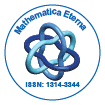# Mathematica EternaOpen Access

ISSN: 1314-3344

## Abstract

### Characterization of ternary semigroups in terms of (∈, ∈ ∨qk) ideals

Anwar Zeb, Gul Zaman, Inayat Ali Shah and Asghar Khan ,

In this paper, we present the concepts of (∈,∈ ∨qk)-fuzzy ideals in ternary semigroups, which is a generalization of the (∈,∈ ∨q) fuzzy ideals of a ternary semigroups. In this regard, we define (∈,∈ ∨qk)-fuzzy left (right, lateral) ideals, (∈,∈ ∨qk)-fuzzy quasi-ideals and (∈,∈ ∨qk)- fuzzy bi-ideals and prove some basic results using these definitions. Special concentration is paid to (∈,∈ ∨qk)-fuzzy left (right, lateral) ideals, (∈,∈ ∨qk)-fuzzy quasi-ideals and (∈,∈ ∨qk)-fuzzy bi-ideals. Furthermore, we characterize regular ternary semigroups in terms of these notions.

Top